# 2018年计算机二级C语言试题及答案(1)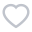632人已考
•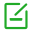卷面总分：100
•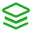试卷类型：预测试题
•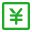测试费用： 免费
•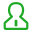关注人数：10325
•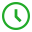作答时间：120分钟
•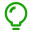解析：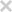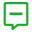试卷简介：
2018年计算机二级C语言试题及答案(1)，是针对计算机二级考试中C语言的预测试题。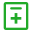试题类型：
• 单选题
• 基本操作题
• 程序改错题
• 程序设计题• 单选题
• 基本操作题
• 程序改错题
• 程序设计题
1

A.外模式

B.内模式

C.概念模式

D.逻辑模式

2

#include<stdio．h>

main（　　）

{ char a，b，C；

b=l；c=A；

for(a=0；a<6；a++)

{ if(a%2)putchar(b+a)；

else putchar(c+a)；

}

}

A.1B3D5F

B.ABCDEF

C.A2C4E6

D.123456

3

A.概念模式

B.内模式

C.外模式

D.数据模式

4

A.整数：1，200

B.实数：1．5E2．0

C.字符斜杠："\"

D.字符串："\007"

5

#include<stdio．h>

main（　　）

{ int a=0，i；

for(i=1；i<5；i++)

{ switch(i)

{ case 0:

case 3:a+=1；

case 1:

case 2:a+=2；

default:a+=3；

}

}

printf("%d"，i)；

}

A.19

B.1

C.6

D.8

6

A.各数据结点的存储空间可以不连续，但它们的存储顺序与逻辑顺序必须一致

B.各数据结点的存储顺序与逻辑顺序可以不一致，但它们的存储空间必须连续

C.进行插入与删除时，不需要移动表中的元素

D.以上3种说法都不对

7

#include<stdio．h>

main（　　）

{char b，C；int i；

b=a；c=A；

for(i=0；i<6；i++)

{if(i％2)putchar(i+b)；

else putchar(i+c)；

}printf("\n")；

}

A.ABCDEF

B.AbCdEf

C.aBcDeF

D.abcdef

8

fun(char*a,char*b) ．

{while((*b=*a)!=\0){a++；b++；})

A.将a所指字符串赋给b所指空间

B.使指针b指向a所指字符串

C.将a所指字符串和b所指字符串进行比较

D.检查a和b所指字符串中是否有\O

9

main（　　）

{int i=1，j=2，k=3；

if(i++= =1(++j= =3= =‖k++= =3))

printf("%d%d%d＼n"，i，J，k)；

)

A.1 2 3

B.2 3 4

C.2 2 3

D.2 3 3

10

#include<stdio．h>

main（　　）

{int m=1，n=2，*p=&m，*q=&n，*r；

r=P；P=q；q=r；

printf("％d，％d，％d，％d\n"，m，n，*P，*q)；

}

A.1，2，1，2

B.1，2，2，1

C.2，1，2，1

D.2，1，1，2